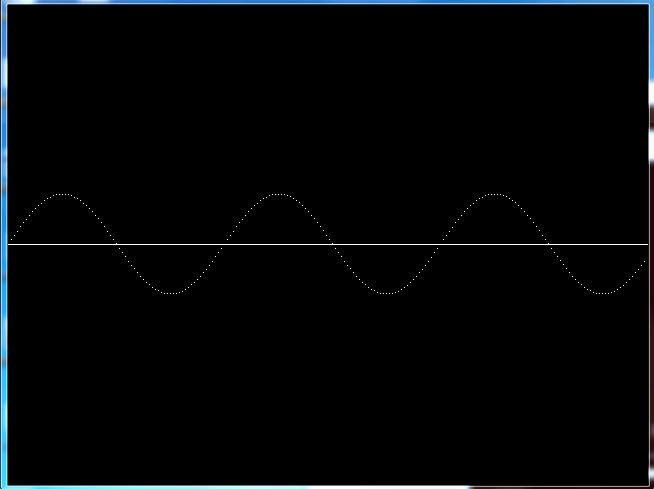# C Program to Draw Sine Wave Using C Graphics

Here is the C graphics program to draw sine wave using graphics.h header file. In this program, we will draw a horizontal sine wave on screen of amplitude 50 pixels. We will use putpixel functions of graphics.h header file to color a pixel at (x, y).

## C program to draw sine wave using graphics

In this program we first initialize graphics mode, by passing graphics driver(DETECT), default graphics mode and specifies the directory path where initgraph looks for graphics drivers (*.BGI). Then we will draw a horizontal axis using line representing the angle in radians. Inside a for loop we calculate the value of cosine for an angle and color the specific pixel using putpixel function. At last we increment the angle by 5.

```#include <math.h>
#include <graphics.h>
#include <dos.h>

int main() {
int gd = DETECT, gm;
int angle = 0;
double x, y;

initgraph(&gd, &gm, "C:\\TC\\BGI");

line(0, getmaxy() / 2, getmaxx(), getmaxy() / 2);
/* generate a sine wave */
for(x = 0; x < getmaxx(); x+=3) {

/* calculate y value given x */
y = 50*sin(angle*3.141/180);
y = getmaxy()/2 - y;

/* color a pixel at the given position */
putpixel(x, y, 15);
delay(100);

/* increment angle */
angle+=5;
}

closegraph();

return 0;
}
```
Program OutputRelated Topics
 C Program to draw cosine(cos) wave graph C Program to draw tangent(tan) graph C Program to draw concentric circles screen C program to draw pie chart using graphics C Program to draw digital counter on screen C graphics program for moving car animation C program for bouncing ball animation List of all C Graphics Programs